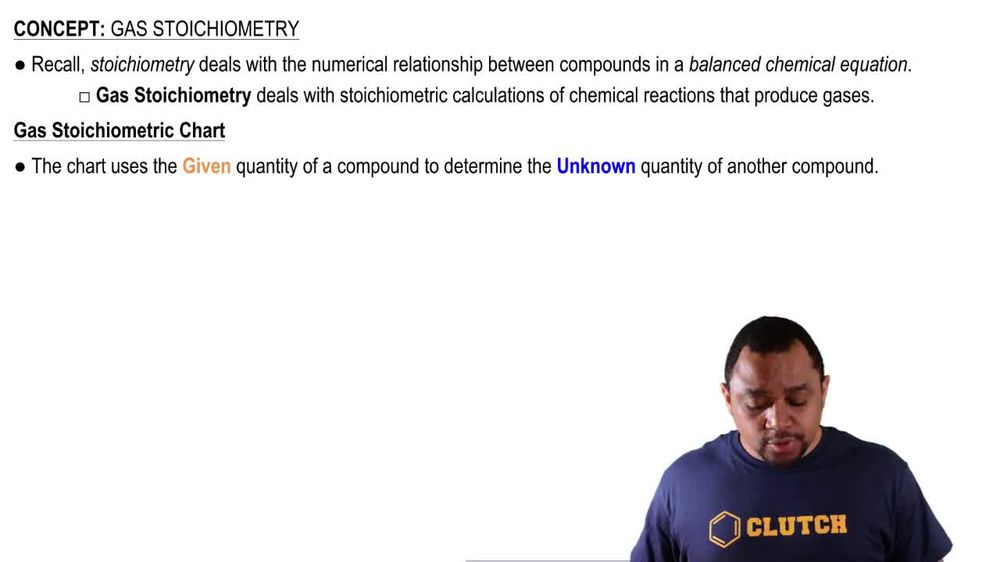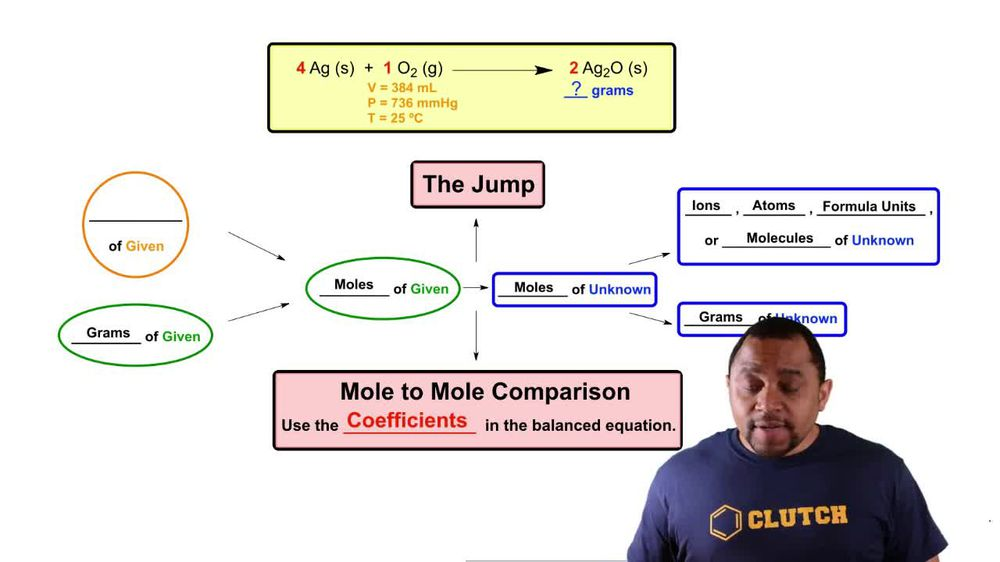Start typing, then use the up and down arrows to select an option from the list.
3:38 minutes
Problem 106a
Textbook Question

# Assume that a single cylinder of an automobile engine has a volume of 524 cm3. (a) If the cylinder is full of air at 74 C and 99.3 kPa, how many moles of O2 are present? (The mole fraction of O2 in dry air is 0.2095.) (b) How many grams of C8H18 could be combusted by this quantity of O2, assuming complete combustion with formation of CO2 and H2O?Verified Solution
This video solution was recommended by our tutors as helpful for the problem above.
71views